Ex 3.1

Chapter 3 Class 7 Data Handling
Serial order wise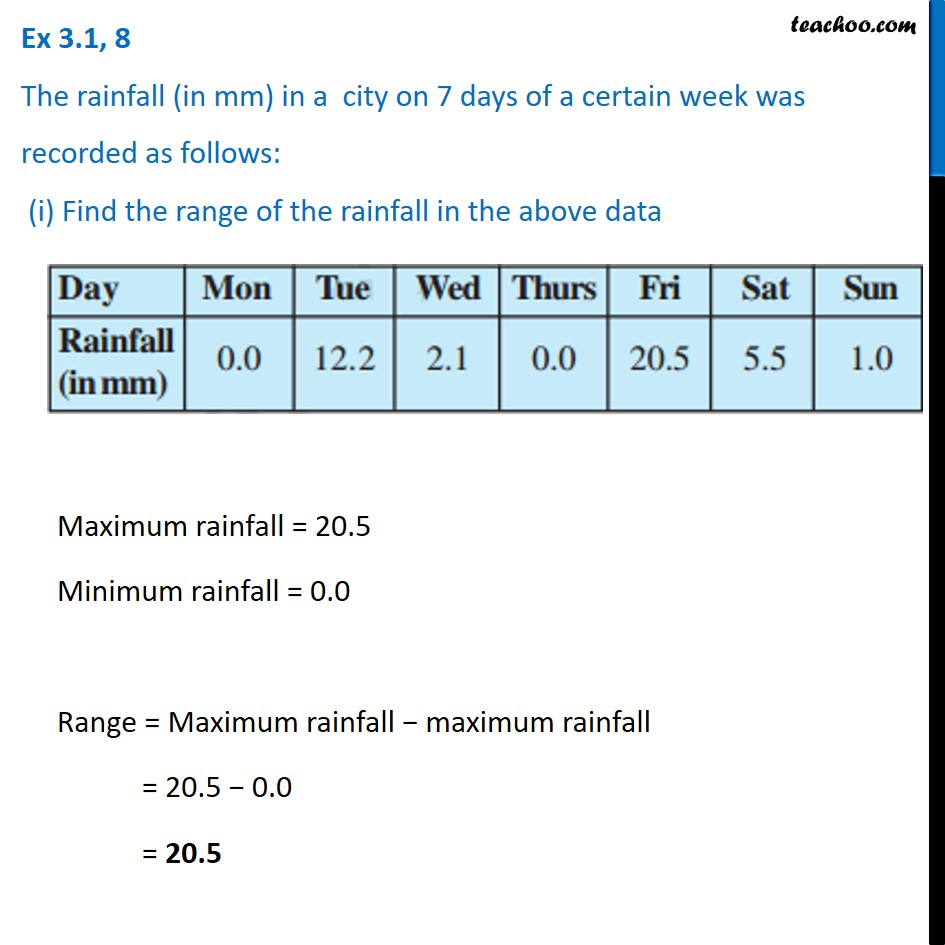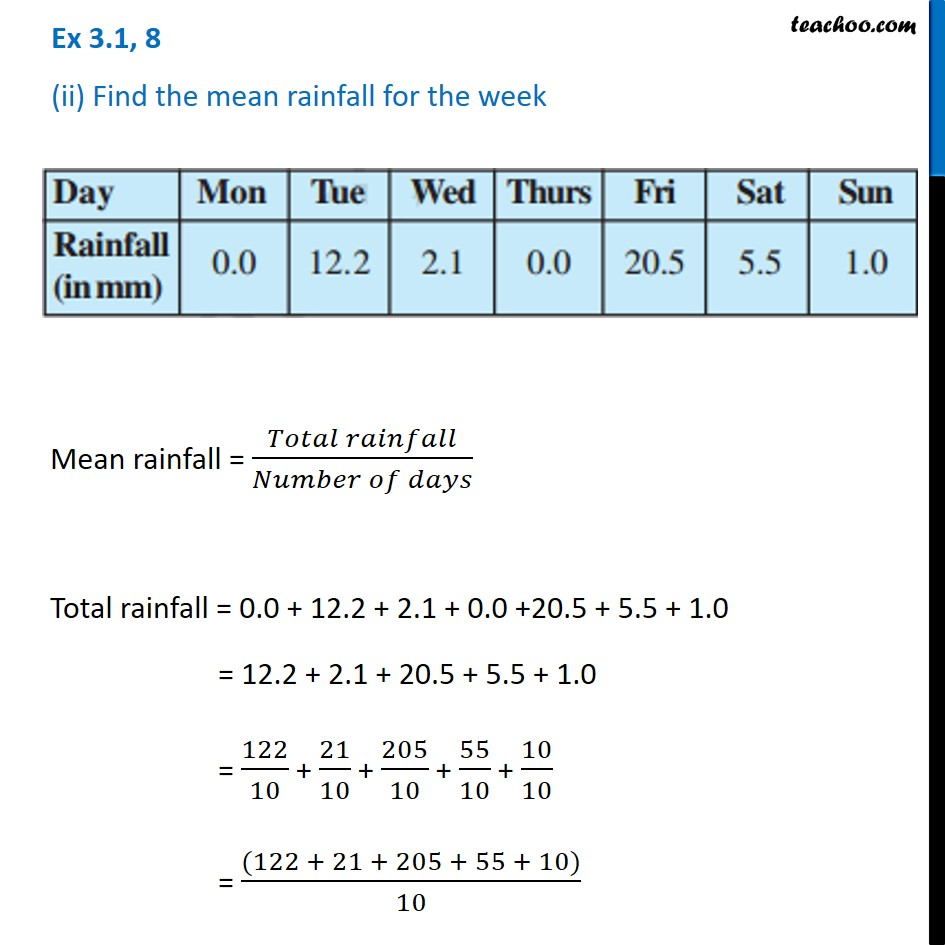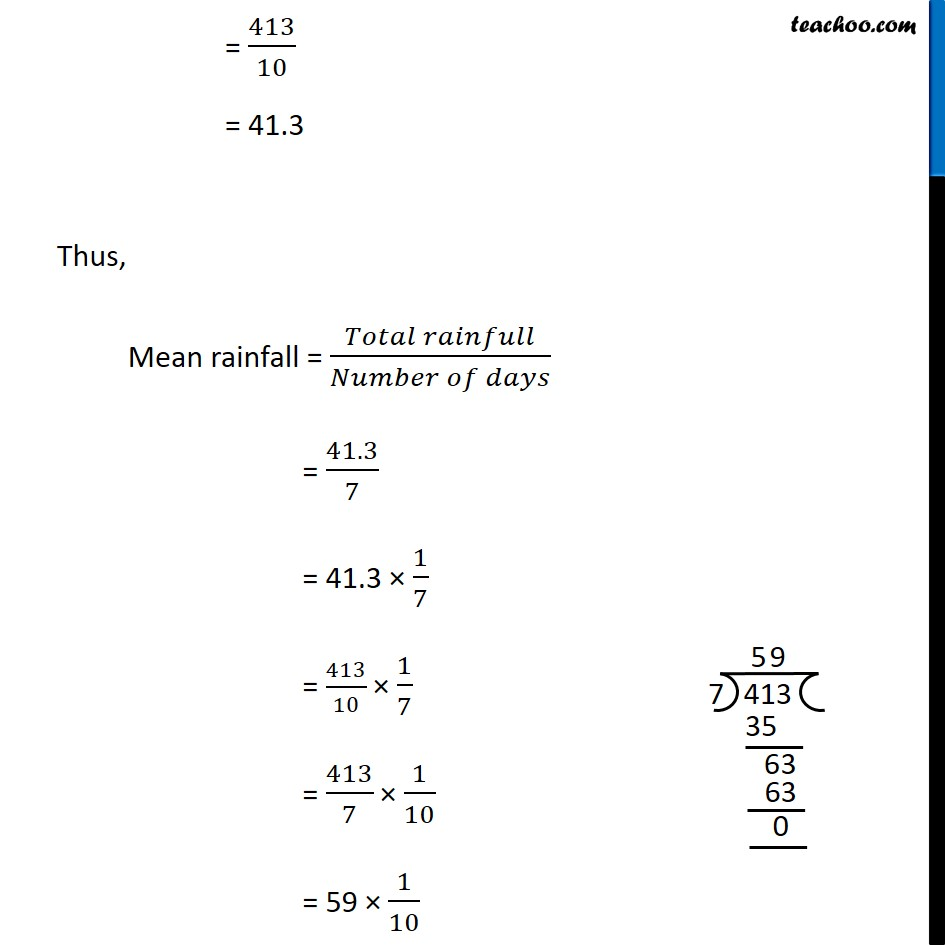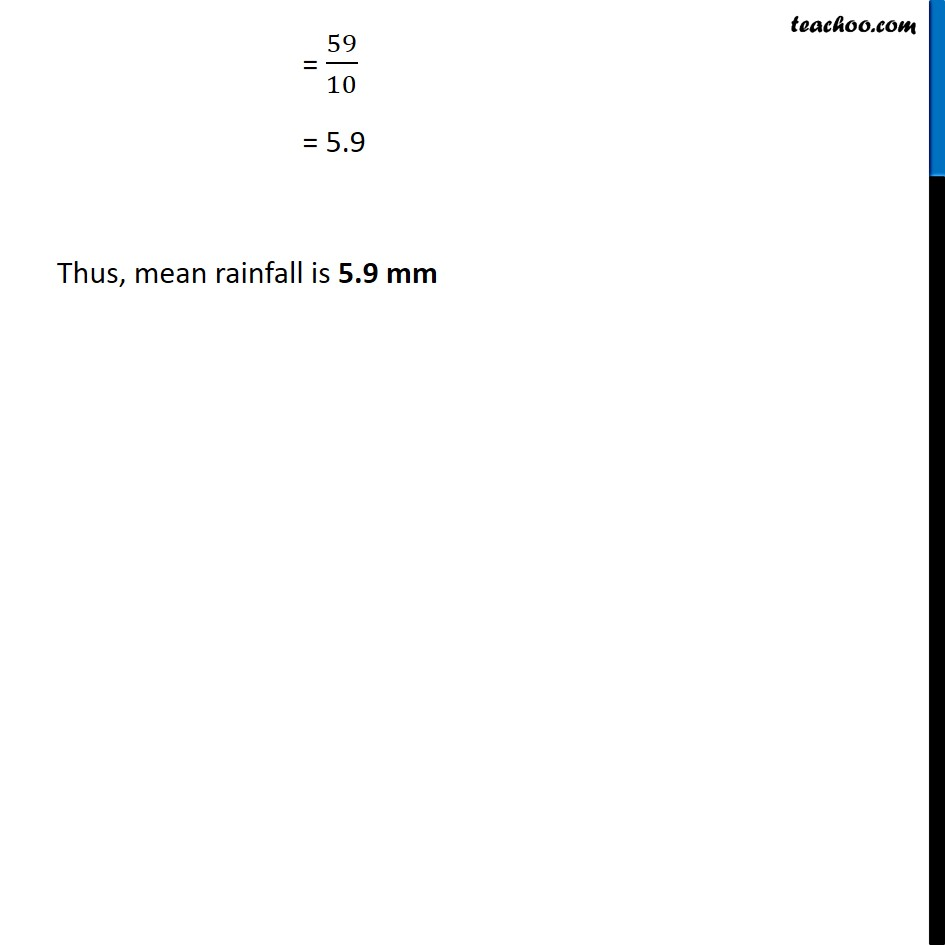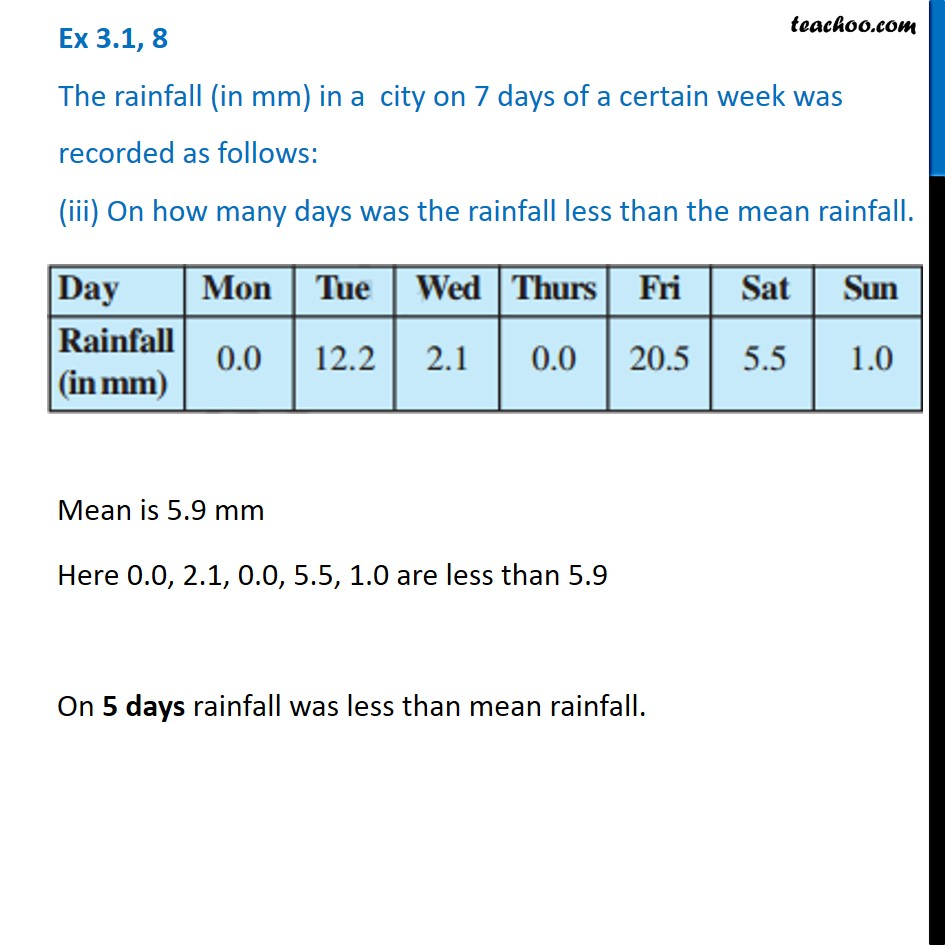Learn in your speed, with individual attention - Teachoo Maths 1-on-1 Class

### Transcript

Ex 3.1, 8 The rainfall (in mm) in a city on 7 days of a certain week was recorded as follows: (i) Find the range of the rainfall in the above data Maximum rainfall = 20.5 Minimum rainfall = 0.0 Range = Maximum rainfall − maximum rainfall = 20.5 − 0.0 = 20.5 Ex 3.1, 8 (ii) Find the mean rainfall for the week Mean rainfall = (𝑇𝑜𝑡𝑎𝑙 𝑟𝑎𝑖𝑛𝑓𝑎𝑙𝑙)/(𝑁𝑢𝑚𝑏𝑒𝑟 𝑜𝑓 𝑑𝑎𝑦𝑠) Total rainfall = 0.0 + 12.2 + 2.1 + 0.0 +20.5 + 5.5 + 1.0 = 12.2 + 2.1 + 20.5 + 5.5 + 1.0 = 122/10 + 21/10 + 205/10 + 55/10 + 10/10 = ((122 + 21 + 205 + 55 + 10))/10 = 413/10 = 41.3 Thus, Mean rainfall = (𝑇𝑜𝑡𝑎𝑙 𝑟𝑎𝑖𝑛𝑓𝑢𝑙𝑙)/(𝑁𝑢𝑚𝑏𝑒𝑟 𝑜𝑓 𝑑𝑎𝑦𝑠) = 41.3/7 = 41.3 × 1/7 = 413/10 × 1/7 = 413/7 × 1/10 = 59 × 1/10 = 59/10 = 5.9 Thus, mean rainfall is 5.9 mm Ex 3.1, 8 (iii) The rainfall (in mm) in a city on 7 days of a certain week was recorded as follows: (iii) On how many days was the rainfall less than the mean rainfall. Mean is 5.9 mm Here 0.0, 2.1, 0.0, 5.5, 1.0 are less than 5.9 On 5 days rainfall was less than mean rainfall.